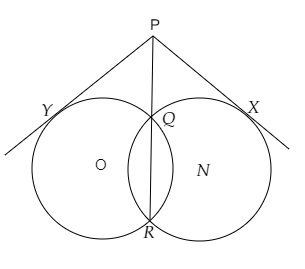# If from any point on the common chord of two intersecting circles, tangents be drawn to the circles, prove that they are equal.

Given:

From any point on the common chord of two intersecting circles, tangents be drawn to the circles.

To do:

We have to prove that they are equal.

Solution:Let $QR$ be the common chord of two circles intersecting each other at $Q$ and $R$.

$P$ be the point on $QR$ when produced.

$PY$ and $PX$ be the tangents drawn to the circles with centres $O$ and $C$ respectively.

Proof:

$PY$ is the tangent and $PQR$ is the secant to the circle with centre $O$.

This implies,

$PY^2 = PQ \times PR$...….(i)

Similarly,

$PX$ is the tangent and $PQR$ is the secant to the circle with centre $C$.

This implies,

$PX^2 = PQ \times PR$..….(ii)

From (i) and (ii), we get,

$PY^2 = PX^2$

$PY = PX$

Hence proved.

Updated on: 10-Oct-2022

65 Views